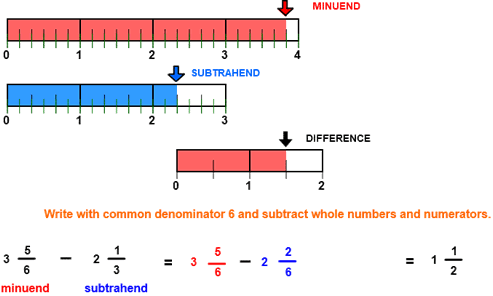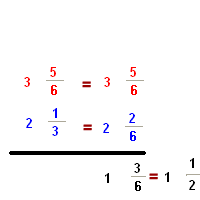# Subtract unlike fractions

## Subtract and Simplify Fractions with Number Line Models

SUBTRACT UNLIKE FRACTIONS
CORRECT:
ATTEMPTS:
SCORE:
PERCENT
WHOLE
NUMERATOR
DENOMINATOR

# INSTRUCTIONS

Follow the directions in the dialog box after pressing the <START> button. The <EXPLAIN> button may be pressed after you enter the subtrahend to see how to do the example.

The following  image was made from Subtract Fractions Designer:The picture shows that the minuend is 3 56 units in length and the subtrahend is 2 13 units in length. The difference can be found by determining the distance from the end of the subtrahend to the end of the minuend.

If a carpenter wants to find the difference in length of two boards, that carpenter will measure from the end of the longer board to the end of the shorter board. You can do the same thing here. Notice that there are 9 spaces from the end of the longer line (minuend) to the end of the shorter line (subtrahend). Each space is 16 of a unit so you would have a difference in length of 96 or 1 12 units.

When the program starts, you will be asked to identify the minuend in the first row and then the subtrahend in the second row. The program will continue when the minuend and subtrahend are correctly identified. You will then be asked to find the difference. The difference must be entered as a whole number or as a mixed number in lowest terms.

You may prefer to work vertically:For more instruction on identifying mixed fractions go to How To Rename From Fraction Form to Mixed Form.

For more instruction on renaming to lower terms go to How To Rename to Lower Terms.

For more instruction on subtracting fractions go to How To Subtract Fractions.

After you enter the difference you may press the <REPORT> button. The report will ask for your name but you may submit a code for your name. This report will give the same results as on the dialog box. The report may be printed or e-mailed.